SAS Namespace Hierarchy and Association Diagrams

# Diagrams for Analysis Metadata Types

The Analysis Hierarchy Diagram depicts the hierarchy of the metadata types that represent statistical transformations, multidimensional data sources, and OLAP information.

Analysis Hierarchy Diagram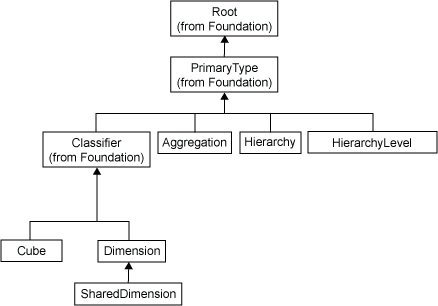The Level and Measure Hierarchy Diagram depicts the hierarchy of the metadata types that represent OLAP information.

Level and Measure Hierarchy Diagram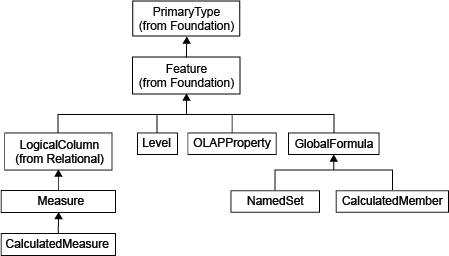The Dimension Associations Diagram illustrates the associations between the Dimension, Level, OLAPProperty, Measure, CalculatedMeasure and Hierarchy metadata types.

Dimension Associations Diagram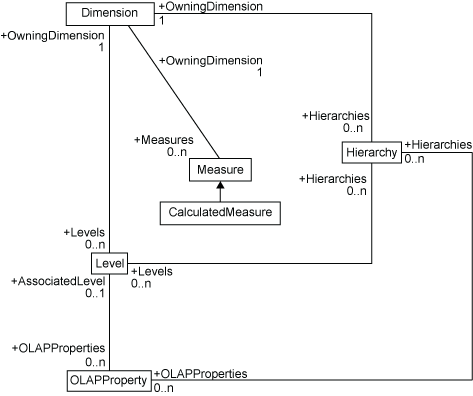The Physical Associations Diagram illustrates the associations between the Cube, File, PhysicalTable, Aggregation, and Level metadata types. The tables from which the Cube is derived are associated via one or more ClassifierMap objects. For more information, see the description of the Transform Submodel.

Physical Associations Diagram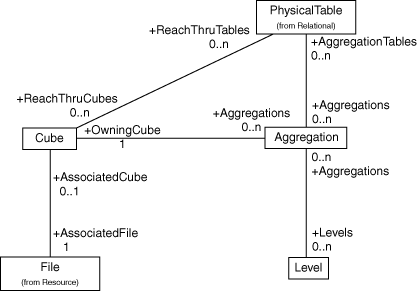The OLAP Schema Diagram illustrates the associations between the DeployedDataPackage, OLAPSchema, Dimension, File, and Cube metadata types.

OLAP Schema Diagram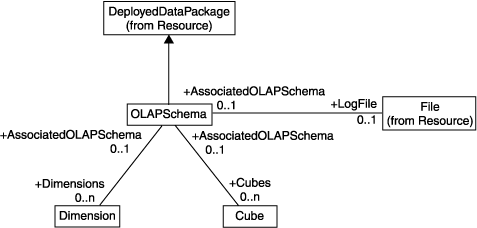The Cube Associations Diagram illustrates the associations between the Cube metadata type and the Measure, Dimension, Hierarchy, and GlobalFormula metadata types.

Cube Associations Diagram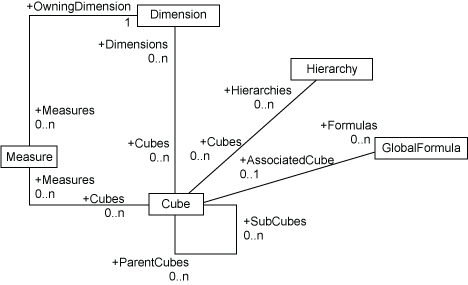The Cube Generation Association Diagram illustrates the Cube generation associations.

Cube Generation Associations Diagram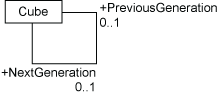The Hierarchy and Level Associations Diagram illustrates the associations between the Hierarchy, Level, and HierarchyLevel metadata types.

Hierarchy and Level Associations Diagram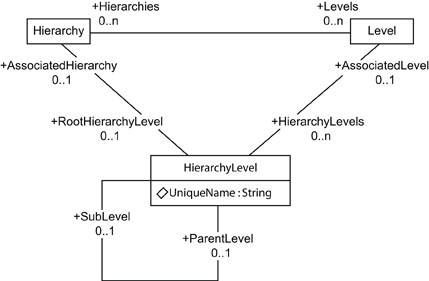The Shared Dimension Associations Diagram illustrates the associations between the OLAPSchema, Dimension, SharedDimension, and Directory metadata types.

Shared Dimension Associations Diagram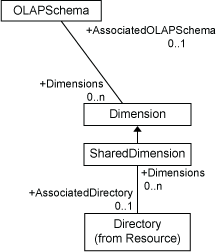The NamedSet and CalculatedMember Associations Diagram illustrates the associations between the Cube, GlobalFormula, CalculatedMember, NamedSet, Hierarchy, and Level metadata types.

NamedSet and CalculatedMember Associations Diagram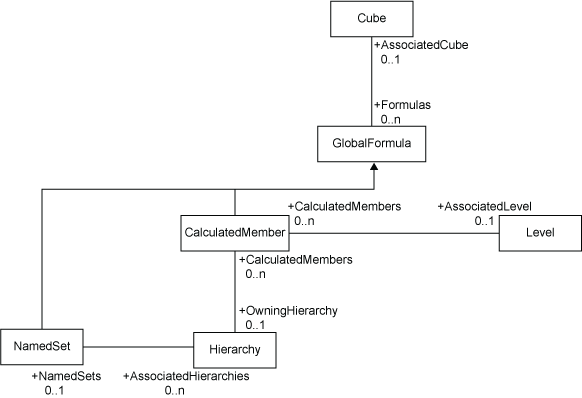Previous Page | Next Page | Top of Page